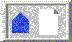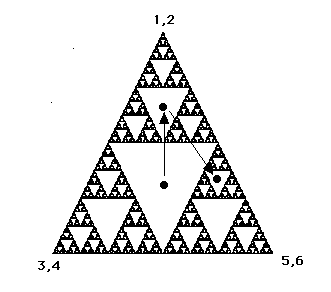Playing the chaos game in (Next Section)Chaos in the Classroom (Cover Page)The Sierpinski triangle. (Previous Section)BU Math Home Page

# Why does the Sierpinski triangle arise from the chaos game?

Students are always intrigued when they first see the Sierpinski triangle emerge from the random chaos game, but there is a simple explanation of why this happens. Suppose we start with a point somewhere in the middle of the largest white (removed) triangle in the Sierpinski triangle.

Where does this point move after one roll of the die? As in Figure 4, we see that this point hops into one of the three next-smaller triangles, since these triangles represent all points that are half the distance to the three vertices from points in the largest removed triangle. After one more iteration, this point then moves to the next smallersize triangle. And so forth.Figure: After one iteration of the chaos game

Now continue. At the next iteration, the point hops into one of the 9 next small triangles, then into the next smaller triangles, and so on. Eventually (after very few iterations), the point enters a small triangle that is for all intents and purposes invisible.

In actuality, the orbit of a point that starts in any of the removed triangles will never ``reach'' the Sierpinski triangle. Rather, it will continue to lie in successively smaller removed triangles. Of course, these removed triangles very quickly become microscopic in size, so for all practical purposes the orbit looks like it lies on S. Mathematicians says that the orbit of the seed is attracted to S. Sometimes S is called a strange attractor.Playing the chaos game in (Next Section)Chaos in the Classroom (Cover Page)The Sierpinski triangle. (Previous Section)BU Math Home Page

Robert L. Devaney
Sun Apr 2 14:31:18 EDT 1995